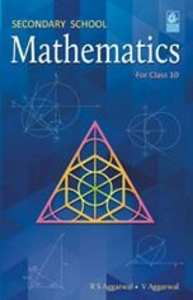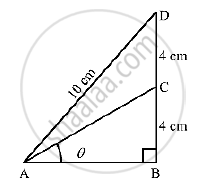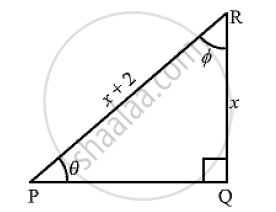# RS Aggarwal solutions for Secondary School Class 10 Maths chapter 5 - Trigonometric Ratios [Latest edition]

#### Chapters## Chapter 5: Trigonometric Ratios

Exercises
Exercises

### RS Aggarwal solutions for Secondary School Class 10 Maths Chapter 5 Trigonometric Ratios Exercises

Exercises | Q 1

If sin θ , sqrt (3)/2 find the value of all T- ratios of θ .

Exercises | Q 2

If cos θ  = 7/25 find the value of all T-ratios of  θ   .

Exercises | Q 3

If tan θ =15/ 8 , find the values of all T-ratios of θ.

Exercises | Q 4

If cot θ =  2 find all the values of all T-ratios of θ .

Exercises | Q 5

If cosec θ = sqrt(10) find all the values of all T-ratios of θ

Exercises | Q 6

If sin θ =  (a^2 - b^2)/(a^2+b^2)find all the values of all T-ratios of θ .

Exercises | Q 7

If 15 cot A = 8 find all the values of sin A and sec A

Exercises | Q 8

If sin A = 9/41 find all the values of cos A and tan A

Exercises | Q 9

If cos θ=0.6 show that (5sin θ -3tan θ) = 0

Exercises | Q 10

If cosec θ= 2 show that (cot θ +sin θ /(1+cos θ )) =2

Exercises | Q 11

If tan θ = 1/sqrt(7) show that   (cosec ^2  θ - sec^2 θ)/(cosec^2 θ + sec^2 θ ) = 3/4

Exercises | Q 12

If tan θ = 20/21 show that ((1-sin θ + cos θ))/((1+ sin θ +cos θ)) = 3/7

Exercises | Q 13

If sec θ = 5/4  show that ((sin θ   - 2 cos θ))/(( tan θ - cot θ)) = 12/7

Exercises | Q 14

If  cot  θ = 3/4 , show that sqrt("sec θ - cosecθ"/"secθ + cosecθ" ) = 1/ sqrt(7)

Exercises | Q 15

If sin θ = 3/4 show that sqrt((cosec^2theta - cot^2theta)/(sec^2theta-1)) =sqrt(7)/3

Exercises | Q 16

If sin θ = a/b,show that (sectheta + tan theta) = sqrt((b+a)/(b-a))

Exercises | Q 17

If cos θ = 3/5 , show that ((sin theta - cot theta ))/(2tan theta)=3/160

Exercises | Q 18

If tan θ = 4/3, show that (sintheta + cos theta )=7/5

Exercises | Q 19

If tan theta = a/b, show that ((a sin theta - b cos theta))/((a sin theta + bcos theta))= ((a^2-b^2))/(a^2+b^2)

Exercises | Q 20

If 3tan θ   4 , show that ((4cos theta - sin theta ))/((4 cos theta + sin theta))=4/5

Exercises | Q 21

If 3 cot theta = 2, show  that ((4 sin theta - 4 cos theta))/((2 sin theta + 6 cos theta ))=1/3

Exercises | Q 22

If 3 cot θ 4 , show that((1-tan^2theta))/((1+tan^2theta)) = (cos^2theta - sin^2theta)

Exercises | Q 23

If sec theta = 17/8  verify that ((3-4sin^2theta)/(4 cos^2theta -3))=((3-tan^2theta)/(1-tan^2theta))

Exercises | Q 24

In the adjoining figure, ∠B  = 90° , ∠BAC = theta° , BC = CD = 4cm and AD = 10 cm. find  (i)  sin theta and (ii) costhetaExercises | Q 25

In a ΔABC , ∠B = 90° , AB= 24 cm and BC = 7 cm find (i) sin A (ii) cos A (iii) sin C (iv) cos C

Exercises | Q 26

In ΔABC , ∠C = 90°  ∠ABC = θ°   BC = 21 units . and AB= 29 units. Show thaT (cos^2 theta - sin^2 theta)=41/841

Exercises | Q 27

In a ΔABC , ∠B = 90° , AB = 12 cm and BC = 5 cm Find
(i) cos A (ii) cosec A (iii) cos C (iv) cosec C

Exercises | Q 28

If sin ∝  = 1/2 prove that (3cos∝ - 4cos^2 ∝)=0

Exercises | Q 29

IF ΔABC ,∠B = 90° AND Tan A = 1/sqrt(3). Prove that

(i) Sin A. cos C+ cos A. Sin c = 1
(ii) cos A. cos C -sin A. sin C = 0

Exercises | Q 30

If ∠A and ∠B are acute angles such that sin A = Sin B prove that ∠A = ∠B.

Exercises | Q 31

If ∠A and ∠B are acute angles such that tan A= Tan B then prove that ∠A = ∠B

Exercises | Q 32

If a right ΔABC , right-angled at B, if tan A=1 then verify that 2sin A . cos A = 1

Exercises | Q 33

In the figure of ΔPQR , ∠P = θ°  and ∠R =∅° find
(i)  sqrt(X +1) cot ∅

(ii)sqrt( x^3 + x ^2) tantheta

(iii) cos θExercises | Q 34

If x = cosec A +cos A and y = cosec A – cos A then prove that (2/(x+y))^2 + ((x-y)/2)^2 = 1

Exercises | Q 35

If x = cot A + cos A and y = cot A – cos A then prove that ((x-y)/(x+y))^2 + ((x-y)/2)^2=1

## Chapter 5: Trigonometric Ratios

Exercises## RS Aggarwal solutions for Secondary School Class 10 Maths chapter 5 - Trigonometric Ratios

RS Aggarwal solutions for Secondary School Class 10 Maths chapter 5 (Trigonometric Ratios) include all questions with solution and detail explanation. This will clear students doubts about any question and improve application skills while preparing for board exams. The detailed, step-by-step solutions will help you understand the concepts better and clear your confusions, if any. Shaalaa.com has the CBSE Secondary School Class 10 Maths solutions in a manner that help students grasp basic concepts better and faster.

Further, we at Shaalaa.com provide such solutions so that students can prepare for written exams. RS Aggarwal textbook solutions can be a core help for self-study and acts as a perfect self-help guidance for students.

Concepts covered in Secondary School Class 10 Maths chapter 5 Trigonometric Ratios are Trigonometry, Trigonometric Ratios, Trigonometric Ratios of Some Special Angles, Trigonometric Ratios of Complementary Angles, Trigonometric Identities, Proof of Existence, Relationships Between the Ratios, Trigonometry, Trigonometric Ratios and Its Reciprocal, Trigonometry, Trigonometric Ratios, Trigonometric Ratios of Some Special Angles, Trigonometric Ratios of Complementary Angles, Trigonometric Identities, Proof of Existence, Relationships Between the Ratios, Trigonometry, Trigonometric Ratios and Its Reciprocal.

Using RS Aggarwal Class 10 solutions Trigonometric Ratios exercise by students are an easy way to prepare for the exams, as they involve solutions arranged chapter-wise also page wise. The questions involved in RS Aggarwal Solutions are important questions that can be asked in the final exam. Maximum students of CBSE Class 10 prefer RS Aggarwal Textbook Solutions to score more in exam.

Get the free view of chapter 5 Trigonometric Ratios Class 10 extra questions for Secondary School Class 10 Maths and can use Shaalaa.com to keep it handy for your exam preparation